EnchantedLearning.com is a user-supported site.
As a bonus, site members have access to a banner-ad-free version of the site, with print-friendly pages.

 You might also like: Mail-Related Words: Little Explorers Picture Dictionary Storytime: Little Explorers Picture Dictionary House-Related Words: Little Explorers Picture Dictionary Pets: Picture Dictionary Money: Little Explorers Picture Dictionary Today's featured page: Periodic Table

 EnglishEnglish-DutchEnglish-FrenchFrench-English English-GermanGerman-EnglishEnglish-ItalianEnglish-JapaneseEnglish-Portuguese English-SpanishSpanish-EnglishEnglish-SwedishEnglish-Phonetic
Little Explorers
English Picture Dictionary
 A B C D E F G H I J K L M N O P Q R S T U V W X Y Z
 Math-Related Words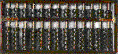abacusAn abacus is an ancient device that is used for arithmetic calculations.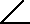acute angleAn acute angle is less than 90 degrees. 1+1=22+3=5additionAddition is the adding of numbers to get their sum.angleAn angle is the amount of rotation it would take to put one intersecting line on top of another. 1+1=27-4=3arithmeticArithmetic is the study of addition, subtraction, multiplication, and division. 1,000,000,000billionA billion is a thousand million. The Earth is billions of years old.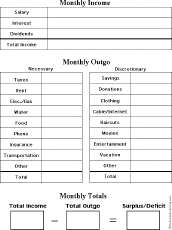budgetA budget is a written account of all income and expenses for a person, a family, an organization, or a government. People use budgets to figure out how much money they take in and plan how much they can spend and save.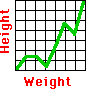chartA chart is a diagram, like a graph or a table, that shows the relationships between things.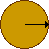circleAll of the points on a circle are the same distance from the center.coneA cone is a shape that has a point at one end and a circular opening at the other end. In mathematics, this shape is a half cone.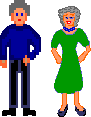coupleA couple refers to two people or two objects.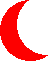crescentA crescent is the shape of the moon a little before of after the time of the new moon.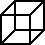cubeA cube is a solid geometric figure with six square faces.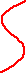curveA curve is a wavy line.cylinderA cylinder is a figure with a long round body .denominatorThe denominator is the bottom number in a fraction.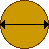diameterThe diameter is the longest distance from one side of a circle (or a sphere) to the other.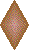diamondA diamond is a four-sided figure whose sides are all the same length. People play baseball on a diamond-shaped field.dividendThe dividend is the number that is divided (in long division). The dividend divided by the divisor is the quotient (plus a remainder).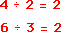divisionDivision is an operation that divides a number into portions.divisorThe divisor is the number that the dividend is divided by (in long division). The dividend divided by the divisor is the quotient (plus a remainder).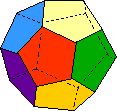dodecahedronA dodecahedron is a twelve-sided geometric solid whose faces are pentagons. 2 xdoubleDouble means twice or two times. ************12dozenDozen is another word for twelve.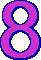eightSpiders have eight legs. Stop signs have eight sides. 18******************eighteenEighteen is the number between seventeen and nineteen. 80eightyEighty is the number between seventy-nine and eighty-one. 11***********elevenEleven is ten plus one.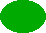ellipseAn ellipse is a flattened circle. =equalWhen two things are equal they are exactly the same in number, quality, or amount.equilateral triangleThe sides of an equilateral triangle are all the same length. 0, 2, 4, 6, 8, 10, ...even numberAn even number is divisible by two.FahrenheitFahrenheit is a measure of temperature that is abbreviated F. Water freezes at 32°F and boils at 212°F.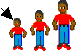firstThe first person or thing comes before the others. 15***************fifteenFifteen is the number after fourteen (14) and before sixteen (16). 50**************************************************fiftyFifty is the number after forty nine (49) and before fifty one (51).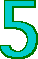fivePeople have five fingers on each hand. 40****************************************fortyForty is the number after thirty nine (39) and before forty one (41).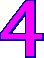fourSquares have four sides. Cats and dogs have four legs. 14**************fourteenFourteen is the number after thirteen (13) and before fifteen (15).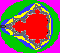fractalFractals are patterns within patterns within patterns. 1/2fractionA fraction is a part of a whole. Half of an apple is a fraction of an apple. The top number in a fraction is called the numerator; the bottom number in a fraction is called the denominator.geometryGeometry is the mathematical study of points, lines, angles, and solids.graphA graph is a diagram that shows relationships between things. >greater thanThe mathematical symbol > means "greater than." When one number is greater than a second number, the first one is bigger than the second. For example, 4 is greater than 2, or 4 > 2.
1/2
half

When something is divided into two equal parts, each of these two parts is half of the original object.heart

The heart is a shape that often symbolizes love.heptagon

A heptagon is a seven-sided figure.hexaflexagon

A hexaflexagon is a folded geometric figure that can be "flexed" to expose its many sides.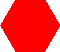hexagon

A hexagon is a six-sided figure. Beehives have hexagonal cells.
100

hundred

One hundred is the number after 99 and before 101.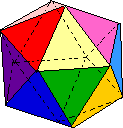icosahedron

An icosahedron is a twenty-sided geometric solid.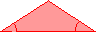isosceles triangle

An isosceles triangle has two sides that are the same length and two angles that are the same.kite

A kite is a four-sided figure in which the two pairs of adjacent sides have the same length.
<

less than

The mathematical symbol < means "less than." When one number is less than a second number, the first one is smaller than the second. For example, 1 is less than 2, or 1 < 2.line segment

A line segment is a portion of a line.long division

In long division, the dividend is divided by the divisor, resulting in a quotient plus a remainder.
 5 4 6 6 5 4 4 6 5

magic square

In a magic square, the rows, columns, and diagonals all add up to the same number.mathematics

Mathematics is the study of numbers, shapes, patterns, and logical reasoning.
1,000,000

million

A million is a thousand thousands. The dinosaurs lived millions of years ago.
-

minus

The mathematical symbol - means "minus." Four minus three is written, 4 - 3.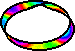Möbius strip

A Möbius strip is a piece of paper with only one side!
1 x 2 = 2

2 x 3 = 6

multiplication

Multiplication is a mathematical operation used to compute areas and do other calculations.
x

multiply

The mathematical symbol x means "multiply." Four times three is written, 4 x 3.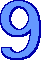nine

Nine is the number between eight and ten. There are nine players on a baseball team.nonagonA nonagon is a nine-sided polygon.number lineNumbers correspond to points on a number line.numberNumbers tell you "how many" or "how much."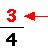numeratorThe numerator is the top number in a fraction.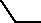obtuse angleAn obtuse angle is greater than ninety degrees.octagonAn octagon is an eight-sided figure. Stop signs are octagons.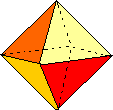octahedronAn octahedron is an eight-sided geometric solid. 1, 3, 5, 7, 9, 11, ...odd numberAn odd number is not divisible by two.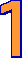oneOne is a small number. 1/2one halfWhen something is divided into two equal parts, each of these two parts is one half of the original object.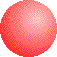orbA ball-shaped object is an orb.ovalAn oval is an egg-shaped figure.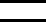parallelParallel lines extend in the same direction, are always the same distance apart, and never meet.parallelogramA parallelogram is a four-sided figure whose opposite sides are parallel.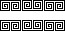patternA pattern is something that is repeated.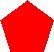pentagonA pentagon is a five-sided figure.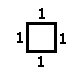perimeterPerimeter is the distance around the edges of a figure. For example, the perimeter of a square with side length of a is a+a+a+a=4 times a.perpendicularWhen two lines are perpendicular, they are at right angles (90 degrees).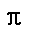piPi is the ratio of the circumference of a circle to the diameter of a circle (this ratio is the same for any size circle). Pi is roughly equal to 3.14159.pie chartA pie chart (also called a circle graph) is a diagram that is useful for displaying information about the percentages or parts of a whole. .pointA point is an exact location, a spot with no width or thickness.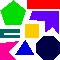polygonA polygon is a many-sided figure with straight edges.polyhedronPolyhedra are solids whose faces are polygons. 2,3,5,7,11,13,17...prime numberA prime number is a positive number that has exactly two factors, 1 and itself. Alternatively, you can think of a prime number as a number greater than one that is not the product of smaller numbers. For example, 13 is a prime number because it can only be divided evenly by 1 and 13. For another example, 14 is not a prime number because it can be divided evenly by 1, 2, 7, and 14. The number one is not a prime number because it has only one factor, 1 itself.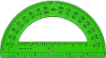protractorA protractor is a device that measures angles.pyramidA pyramid is a shape that has a flat polygonal base and triangular sides that meet at a point on the top. The pyramids in Egypt are huge buildings build by ancient Egyptians.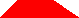quadrilateralA quadrilateral is a four-sided figure. The square, rectangle, rhombus, trapezoid, kite, and parallelogram are quadrilaterals.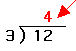quotientThe quotient is the answer in long division. The dividend divided by the divisor is the quotient (plus a remainder).radiusThe radius is the distance from the center of a circle (or a sphere) to the edge. 1/2ratioA ratio is the relationship between two numbers, as defined by the result you get when you divide one by the other.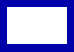rectangleA rectangle is a four-sided figure whose sides are at right angles to each other.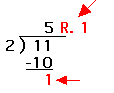remainderThe remainder is the fractional part of the answer in long division. The dividend divided by the divisor is the quotient plus the remainder.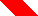rhombusA rhombus is a parallelogram with equal-length sides.right angleA right angle looks like the corner of a square; it extends ninety degrees.right triangleA right triangle has one angle that is a right angle (extending 90 degrees).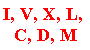Roman numeralsThousands of years ago, in ancient Rome, the Romans used a system of numbers that we call Roman numerals. In this system, I=1. V=5, X=10, L=50, C=100, D=500, and M=1,000.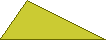scalene triangleThe sides of a scalene triangle are all different lengths.secondThe second person or item comes after the first and before the third.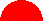semicircleA semicircle is half a circle.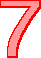sevenThere are seven days in the week.shapesEverything has a shape.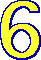sixInsects have six legs.sphereA sphere is a ball-shaped object.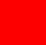squareA square has four, equally long sides which are at right angles to each other.starA star is a shape that has many points. 1-1=05-3=2subtractionSubtraction is the operation of taking one number away from another.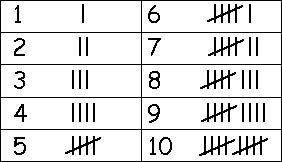tally marksTally marks are a quick way of keeping track of numbers in groups of five. One vertical line is made for each of the first four numbers; the fifth number is represented by a diagonal line across the previous four.tenPeople have ten fingers and ten toes.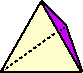tetrahedronA tetrahedron is a pyramid formed by four triangles.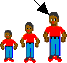thirdThe third person or item comes after the first and second. 13*************thirteenThirteen is the number after twelve (12) and before fourteen (14). 30******************************thirtyThiry is the number after twenty nine (29) and before thirty one (31). 1,000thousandOne thousand is a whole number that is equal to 100 times 10.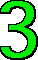threeThree is the number between 2 and 4. A tricycle has three wheels. A triangle has three sides. A Triceratops has three horns. Tri means three!torusA torus is a doughnut-shaped object.trapezoidA trapezoid is a four-sided figure with exactly two parallel sides.triangleA triangle is a geometric figure that has three sides. 12************twelveTwelve (12) is the number between eleven (11) and thirteen (13). Dozen is another word for twelve.twentyThere are twenty (20) apples above.twoWe have two eyes, two ears, two arms, and two legs. 1 + 1 ≠ 3unequalTwo things are unequal if they are not the same.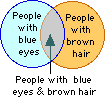Venn diagramA Venn diagram is a diagram that uses overlapping circles to show relationships among sets of things. 0zeroZero is a number that stands for the absence of size or quantity.

 Little ExplorersEnglish Picture Dictionary
 A B C D E F G H I J K L M N O P Q R S T U V W X Y Z
 Adjectives Adverbs Astronomy Bathroom Beach Birds Birthday The Body Buildings Camping City Clothing Colors Containers Devices Farm Firehouse Flowers Food Furniture Geology House Location Mail Math Measurement Money Music People Pets Prepositions Plants Punctuation School Sea Shapes Sports Storytime Time Tools Toys Verbs Vehicles Weather Winter Words

Enchanted Learning®
Over 35,000 Web Pages
Sample Pages for Prospective Subscribers, or click below

 Overview of Site What's New Enchanted Learning Home Monthly Activity Calendar Books to Print Site Index K-3 Crafts K-3 Themes Little ExplorersPicture dictionary PreK/K Activities Rebus Rhymes Stories Writing Cloze Activities Essay Topics Newspaper Writing Activities Parts of Speech Fiction The Test of Time iPhone app TapQuiz Maps - free iPhone Geography Game Biology Animal Printouts Biology Label Printouts Biomes Birds Butterflies Dinosaurs Food Chain Human Anatomy Mammals Plants Rainforests Sharks Whales Physical Sciences: K-12 Astronomy The Earth Geology Hurricanes Landforms Oceans Tsunami Volcano Languages Dutch French German Italian Japanese (Romaji) Portuguese Spanish Swedish Geography/History Explorers Flags Geography Inventors US History Other Topics Art and Artists Calendars College Finder Crafts Graphic Organizers Label Me! Printouts Math Music Word Wheels

Enchanted Learning Search

 Search the Enchanted Learning website for: